×

Search anything:

# Deep Learning Practice Questions

#### Deep Learning List of Interview QuestionsIn this article, we will be going over 50 practice questions related to deep learning.

# General Terminology and Concepts

1. What is a neural network?

A neural network, also known as an artificial neural network (ANN), is the foundation of deep learning algorithms. It is inspired by the structure of the human brain. The basic unit in a neural network is a neuron, and neurons are organized into a series of interconnected layers that can send and receive information.

1. What is an input layer?

The input layer is the first layer in a neural network. It takes input values and passes them on to the next layers for processing.

1. What is an output layer?

The output layer is the last layer of the neural network and produces the network's outputs.

1. What are hidden layers?

Hidden layers are the layers between the input and output layers. This is where all the computation and "learning" in a neural network is done. Usually, each layer learns different aspects about the data in order to minimize the error or cost function.

1. What is a weight?

A weight is a parameter in a neural network that controls the strength of the connection between two neurons. When a neuron receives an input, it multiplies this input by the corresponding connection's weight.

1. What is a bias?

A bias is a constant that offsets the result of the inputs multiplied by their corresponding weights. After the inputs are multiplied by the weights of the corresponding connection, the bias value is added. Bias is analogous to the constant in a linear equation..

1. What is an activation function?

After the weighted sum of the inputs is calculated, the activation function transforms it into an output that is sent to the next neurons.

1. Which of the following is not an activation function?

A. Sigmoid
B. ReLU
C. Dot Product
D. tanh

Explanation: Sigmoid, ReLU, and tanh are all activation functions. A dot product is not an activation function.

1. What is the ReLU activation function?

The rectified linear unit (ReLU) activation function is a piecewise function that outputs 0 if the input is negative and the original input if it is positive. Mathematically, this can be represented as the following equation: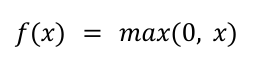1. What is the sigmoid activation function?

The sigmoid function is a special form of the logistic function. Its domain includes all real numbers, and its output is always between 0 (exclusive) and 1 (exclusive). Its graph is shaped like an elongated "S", and the equation below represents the sigmoid function.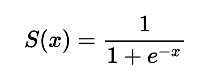1. What is the softmax activation function?

Softmax is an activation function that converts a vector of numbers into a vector of probabilities. These probabilities add up to one. The softmax function is commonly used as the activation function in the output layer of neural networks for multiclass classification problems.

1. What is a GPU and why is it used?

A Graphics Processing Unit (GPU) is a specialized processor that was originally designed to speed up graphics rendering. It is commonly used in deep learning since it can perform simultaneous computations and can process more data than a Central Processing Unit (CPU).

1. What is a GAN?

A Generative Adversarial Network (GAN) is a type of generative model, meaning that it can create new data instances resembling samples in your training data. A GAN's structure consists of two parts: a generator that generates new data, and a discriminator that learns to distinguish real data from synthetic data. Essentially, the generator tries to fool the discriminator, and the discriminator tries to not be fooled.

# How models learn

1. What does the learning rate of a model represent?

As a model learns, its weights and biases are updated so that it can minimize the cost or error function. Learning rate is a hyperparameter that controls how much the model's weights are changed every time in response to the estimated error.

The goal of a neural network, while it is training, is to minimize a cost function. Gradient descent is an iterative optimization algorithm used to find a local minimum of the cost function.

1. What is backpropagation?

Gradient descent needs to calculate derivatives to determine which direction to move in to find a local minimum. Backpropagation is the process of calculating these derivatives. The neural network's error is calculated once the inputs have propagated forward to the output layer. From this output layer, the network error propagates backwards to the input layer, a process called backpropagation. This helps calculate how much the weights of each node need to change in order to reduce the error.

1. Do neural networks require manual feature extraction?

Neural networks do not require you to extract features. They can operate on raw data and learn complex features themselves.

1. Which of the following techniques can be used to prevent overfitting in a neural network?

A. Dropout
B. Early Stopping
C. Data Augmentation
D. All of the Above

Explanation: Dropout, early stopping, and data augmentation are all techniques used to prevent overfitting. Dropout works by randomly disabling neurons and their connections, and this prevents the neural network from relying too much on any single neuron. Early stopping is a method that stops training a neural network when its performance on the validation data stops improving. Data augmentation is a technique used to increase the amount of training data and usually causes the neural network to generalize better, thus reducing overfitting.

# Computer Vision and Convolutional Neural Networks

1. What is a convolution?

A convolution is performed on the input data using a kernel, also called a filter, to produce a feature map. A convolution is executed by ‘sliding’ the kernel over the input, and the dot product goes into the feature map. In the below image, there is a sample input image’s pixel values. On the right, there is a kernel that will be applied to the input image.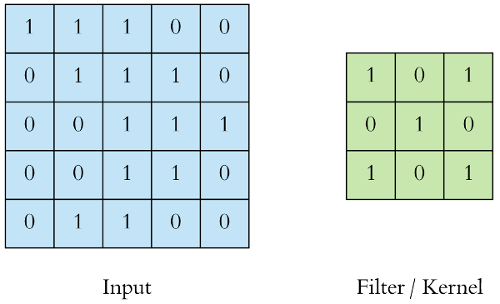The kernel, or filter, slides across the input image. Each weight is multiplied by the corresponding input pixel, and the sum of these multiplications goes into the feature map.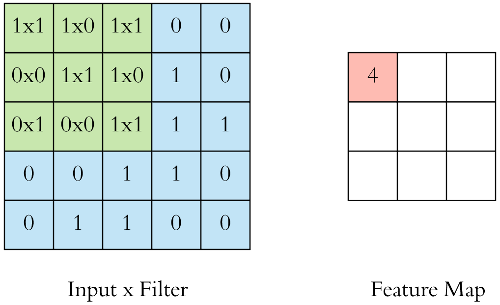1. What is a filter and what does it do?

A filter is a matrix of weights that are used to extract features during a convolution. The individual values in the filter are updated during the training process.

1. What is a receptive field?

The area of the original image that the filter covers is called the receptive field.

1. What is a dot product?

A dot product is the sum of the element-wise multiplication between the receptive field of the input and filter. This results in a scalar value, and for this reason, a dot product is sometimes also called a scalar product.

1. What is pooling?

Pooling reduces the dimensionality of feature maps that are generated by convolutions. This reduces the amount of computation that needs to be done but it still retains the significant information, therefore decreasing training time without significant accuracy loss. This is done using a filter that slides across the feature map and represents each individual region with one number. Depending on the type of pooling used, the number used will vary.

1. Explain max pooling.

Max pooling is when the greatest number in each window is used, as shown below.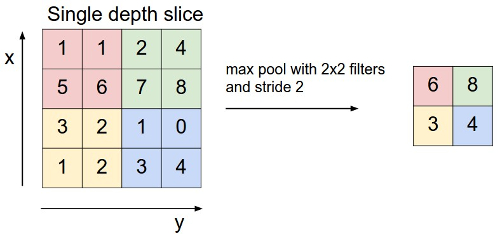1. Explain min pooling.

Min pooling is when the lowest number in each window is used to represent that region of the feature map.

1. Explain average pooling.

Average pooling represents each region of the feature map by calculating its average value.

1. Which of the following are layers in a Convolutional Neural Network?

A. Convolutional Layers
B. Pooling Layers
C. Fully-connected Layers
D. All of the Above

Explanation: Convolutional layers, pooling layers, and fully-connected layers are all layers used in a neural network. A convolutional layer is the main building block of a CNN. It contains the filters, or kernels, that convolve within the image. The pooling layer typically comes after the convolutional layer. Fully connected layers form the last layers of the neural network, and they come after the final convolutional or pooling layer.

1. Which activation function does a CNN typically use?

A Convolutional Neural Network typically uses the Rectified Linear Unit (ReLU) activation function.

# Natural Language Processing (NLP)

1. What are some common applications of natural language processing?

Natural language processing (NLP) is used for text classification (as in spam filtering or intent classification), machine translation (e.g. Google Translate), text summarization, question answering (extracting key information from text to answer a question), and much more.

1. What are word embeddings?

Word embeddings are a way to numerically represent words as real-valued vectors. Word embeddings allow words with similar meanings to have a similar representation. Word embeddings are also able to learn relationships between words. For example, the difference between the word embeddings of the words "woman" and "man" is about the same as the difference between the words "aunt" and "uncle".

1. List some common word embedding techniques.

Word embedding techniques include Google's word2vec, Stanford's GloVe, and TF-IDF.

1. Which Python library offers an implementation of the word2vec model?

Gensim

1. Which Python library offers an implementation of the GloVe model?

glove_python

1. What is an RNN and why is it used in NLP?

A Recurrent Neural Network (RNN) is a type of neural network used to deal specifically with sequences of data. It works well with sequential data because of its internal memory. For example, let's take a look a simple text classification problem. In natural language, the order of the words and the context surrounding those words are used by RNNs, unlike several other approaches. The RNN takes the output of the first word and feeds it into the output of the second word, and this process continues. Every word uses the output of the previous word to help make a prediction. That is why RNNs are used for sequential data, including text classification, named-entity recognition, and time series data.

1. What is an LSTM?

Long short-term memory (LSTM) networks are a special type of RNN capable of learning long-term dependencies. LSTMs overcome two technical problems - vanishing gradients and exploding gradients, both of which are related to training.

# Basics of TensorFlow

1. What is TensorFlow?

TensorFlow is an open source platform for machine learning that allows developers to create, train, test, and deploy machine learning models, including several types of neural networks. It works with Python, Java, and C++., and can be also used in the browser, mobile applications, and even Raspberry Pi.

1. What is a tensor?

A tensor are immutable, multi-dimensional arrays with a uniform type. They are similar to NumPy arrays.

1. What is the rank of a tensor?

The rank of a tensor is the number of indices required to select an individual element of the tensor. You can think about it as the number of dimensions.

1. What is a "rank-0" tensor?

A "rank-0" tensor is one with a single value and no axes.

1. What is another name for a "rank-0" tensor?

A "rank-0" tensor is also called a "scalar".

1. How would you implement a "rank-0" tensor in Python?
``````import tensorflow as tf
rank_0_tensor = tf.constant(52)
print(rank_0_tensor)
``````
1. What is a "rank-1" tensor?

A "rank-1" tensor is like a list of values. It has one axis, or dimension.

1. What is another name for a "rank-1" tensor?

A "rank-1" tensor is also called a "vector".

1. How would you implement a "rank-1" tensor in Python?
``````import tensorflow as tf
rank_1_tensor = tf.constant([14, 1, -1])
print(rank_1_tensor)
``````
1. What is a "rank-2" tensor?

A "rank-2" tensor is a tensor with two dimensions, or axes. You can think of this is a two-dimensional array or a table of values.

1. What is another name for a "rank-2" tensor?

A "rank-2" tensor is commonly referred to as a "matrix".

1. How would you implement a "rank-2" tensor in Python?
``````import tensorflow as tf
rank_2_tensor = tf.constant([[1, 2, 3],
[4, 5, 6],
[7, 8, 9]])
print(rank_2_tensor)
``````
1. What does the shape of a tensor signify?

A tensor's shape is the number of elements in each of its dimensions. For example, a tensor with 2 rows and 3 columns has shape `[2, 3]`

1. How can you determine the number of axes of a tensor in TensorFlow?

You can use the tensor's `ndim` attribute to output the number of dimensions.

``````print(rank_2_tensor.ndim)
``````
1. What is the size of a tensor?

The size of a tensor is the total number of items it contains. This can be calculated by multiplying each element in the tensor's shape vector.

1. How do you calculate the size of a tensor in TensorFlow?

TensorFlow provides a `size()` method that can be used as follows:

``````print(int(tf.size(rank_2_tensor)))
``````
1. How do you convert a tensor to a NumPy array?

There are two methods that can convert a tensor to a NumPy array - `np.array` and `tensor.numpy`:

``````import numpy as np
np.array(rank_2_tensor)
``````
``````rank_2_tensor.numpy()
``````
1. How do you add tensors?

You can use the `tf.add` method or you can use the `+` operator:

``````import tensorflow as tf
``````
``````print(rank_2_tensor + rank_2_tensor)
``````
1. How do you perform element-wise multiplication on tensors?

You can use the `tf.multiply` method or you can use the `*` operator:

``````import tensorflow as tf
print(tf.multiply(rank_1_tensor, rank_1_tensor))
``````
``````print(rank_1_tensor * rank_1_tensor)
``````
1. How do you multiply matrices in TensorFlow?

You can use the `tf.matmul` method or you can use the `@` operator:

``````import tensorflow as tf
print(tf.matmul(rank_2_tensor, rank_2_tensor))
``````
``````print(rank_2_tensor @ rank_2_tensor)
``````

With this article at OpenGenus, you must have a good practice of basic Deep Learning questions. Enjoy.

#### Reyansh Bahl

Reyansh has been a Machine Learning Developer, Intern at OpenGenus. He is pursing his High School Diploma from North Carolina School of Science and Mathematics in Computer Science.

Deep Learning Practice Questions